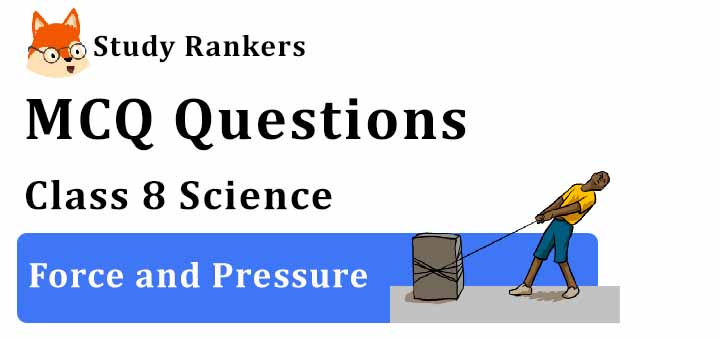## MCQ Questions for Class 8 Science: Ch 11 Force and Pressure1. Force acting on per unit area is called
(a) non-contact forces
(b) contact forces
(c) force
(d) pressure
► (d) pressure

2. The strength of a force is usually expressed by its
(a) Altitude
(b) Duration
(c) Direction
(d) Magnitude
► (d) Magnitude

3. When two forces act in opposite directions, then net force acting is the
(a) Sum of two forces
(b) Difference between two forces
(c) Both of these
(d) None of these
► (b) Difference between two forces

4. Which of the following actions describe pushing force?
(a) Kicking
(b) Lifting
(c) Picking
(d) Opening
► (a) Kicking

5. Magnetic force is
(a) Contact force
(b) Non-contact force
(c) both (a) and (b)
(d) None
► (b) Non-contact force

6. Force is a
(a) Pull
(b) Push
(c) Pull and push both
(d) None of these
► (c) Pull and push both

7. Which one is an example that shows that force can change shape and size of object?
(a) Force applied to increase the speed
(b) Force applied on cricket ball
(c) Force applied on large stone
(d) Force applied on inflated balloon
► (d) Force applied on inflated balloon

8. The force exerted by a charged body on another charged or uncharged body is known as
(a) Magnetic force
(b) Gravitational force
(c) Electrostatic force
(d) Muscular force
► (c) Electrostatic force

9. Which of the following examples describe the change of shape on applying force?
(a) A ball being kicked
(b) A fan switched on
(c) A man jumping from a height
(d) Repeatedly tearing of a paper
► (d) Repeatedly tearing of a paper

10. A force applied on an object may change its
(a) Colour
(b) Mass
(c) Weight
(d) Speed
► (d) Speed

11. Four forces are acting on a body. If the body does not change its position or shape/then what does it mean?
(a) Forces are similar acting in the same direction.
(b) Forces are parallel and opposite.
(c) Forces add up to zero when taken as vectors.
(d) Forces are different acting in the same direction.
► (c) Forces add up to zero when taken as vectors.

12. A push or pull on an object is called
(a) Gravitation
(b) Pressure
(c) Push-pull
(d) Force
► (d) Force

13. How does the pressure exerted by a liquid change?
(a) Increases with depth
(b) Decreases with depth
(c) Remains constant
(d) First increases and then decreases
► (a) Increases with depth

14. A force is applied on an object in the direction of its motion. What is the effect of force on the object?
(a) The speed of the object will increase.
(b) The speed of the object will decrease.
(c) The speed of the object will remain unchanged.
(d) The object comes to rest.
► (a) The speed of the object will increase.

15. Leaves or fruits fall on the ground due to
(a) Magnetic force
(b) Gravitational force
(c) Electrostatic force
(d) Muscular force
► (b) Gravitational force

16. Which of the following effects is caused by frictional force?
(a) Change in the shape of an object
(b) Change in the direction of the motion of an object
(c) Slowing down of a moving object
(d) Acceleration in a moving body
► (c) Slowing down of a moving object

17. State of motion is described by
(a) Position of rest
(b) Position of motion
(c) Both by the state of rest or motion
(d) None of these
► (c) Both by the state of rest or motion

18. Force of gravity is a
(a) Repulsive force
(b) Attractive force
(c) Contact force
(d) Neither attractive nor repulsive force
► (b) Attractive force

19. Force applied on an object in same direction
(a) Divide on another
(c) Multiply on another
(d) Subtract to one another
► (b) Add to one another

20. What causes the atmospheric pressure?
(a) The sky above our head
(b) The air surrounding the earth
(c) The gravitational force of the sun and other planets
(d) The mass of the earth
► (b) The air surrounding the earth

21. A When does a body float on water?
(a) When no force is acting on it.
(b) When the net force acting on the body is zero
(c) When there is a gravitational pull.
(d) When there is friction between the body and the water
► (b) When the net force acting on the body is zero

22. If no force acts on a body at rest, then what effect can be observed?
(a) The body changes shape.
(b) The body moves with increased speed.
(c) The body remains at rest.
(d) The body breaks up.
► (c) The body remains at rest.

23. Which of the following is a non-contact force?
(a) Muscular force
(b) Electrostatic force
(c) Elastic spring force
(d) Frictional force
► (b) Electrostatic force

24. Gravitational force is acting on
(a) Only on moon
(b) Only on other planets
(c) Only on earth
(d) All bodies
► (d) All bodies

25. The tendency of a body to maintain its state of rest or uniform motion is called
(a) Gravity
(b) Inertia
(c) Weight
(d) Acceleration
► (b) Inertia